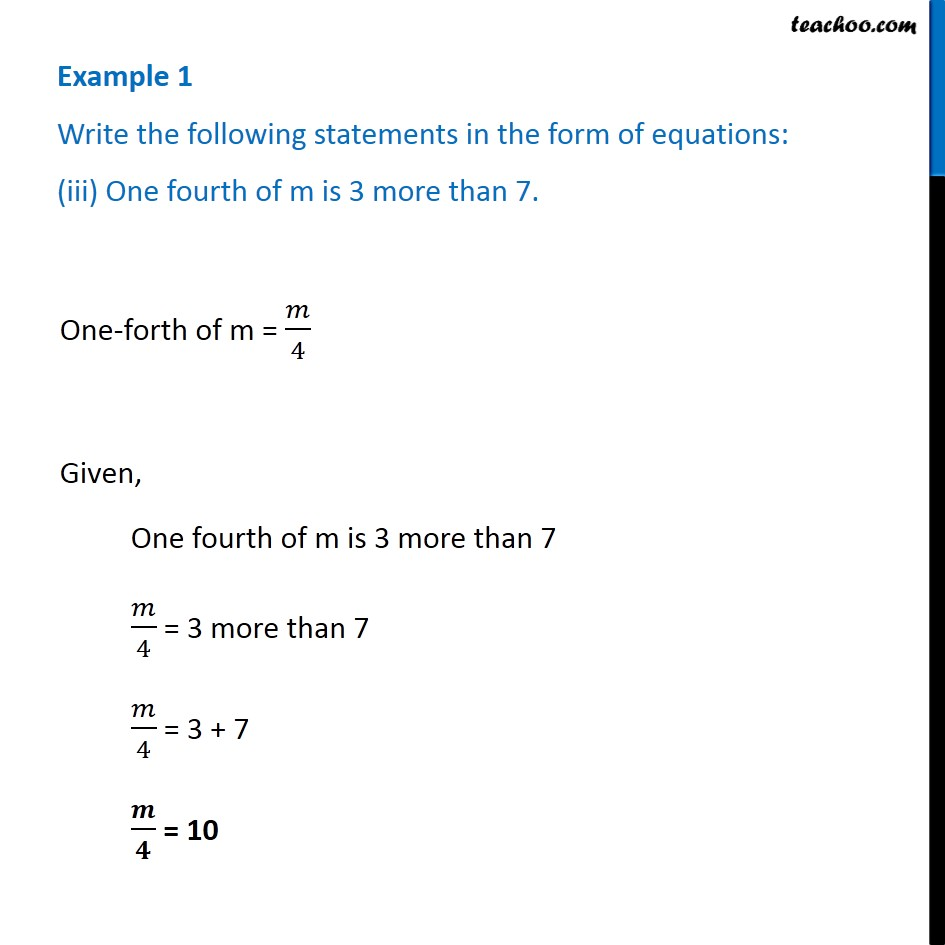Examples

Chapter 4 Class 7 Simple Equations
Serial order wiseLearn in your speed, with individual attention - Teachoo Maths 1-on-1 Class

### Transcript

Example 1 Write the following statements in the form of equations: (iii) One fourth of m is 3 more than 7. One-forth of m = 𝑚/4 Given, One fourth of m is 3 more than 7 𝑚/4 = 3 more than 7 𝑚/4 = 3 + 7 𝒎/𝟒 = 10